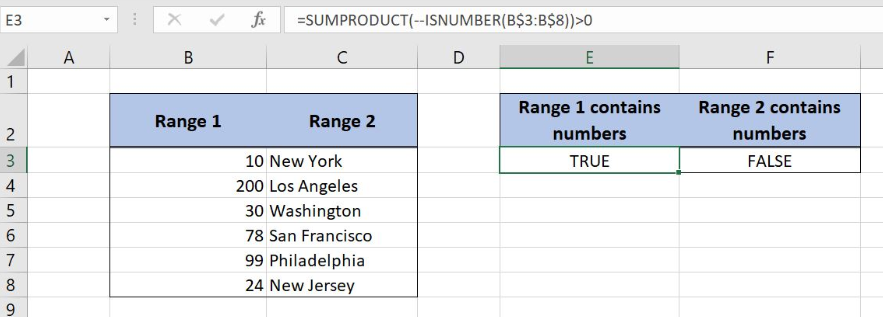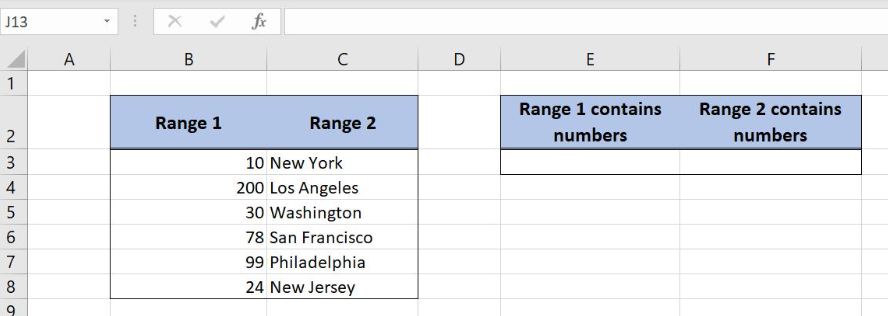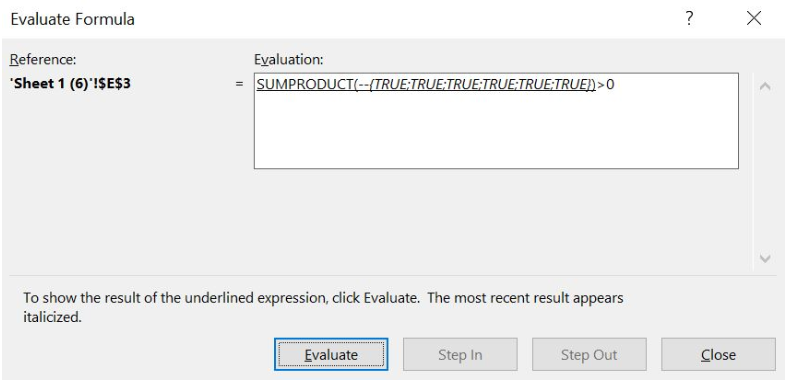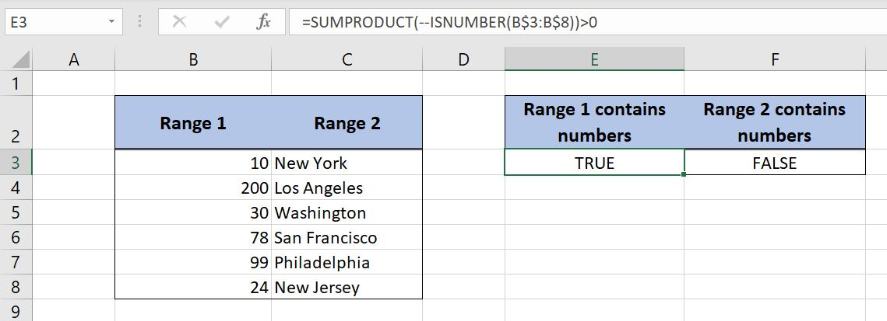Get instant live expert help with Excel or Google Sheets“My Excelchat expert helped me in less than 20 minutes, saving me what would have been 5 hours of work!”

#### Post your problem and you'll get expert help in seconds

Your message must be at least 40 characters
Our professional experts are available now. Your privacy is guaranteed.

# Range contains numbers

Excel allows a user to check if a range contains numbers, by using the SUMPRODUCT and ISNUMBER functions. As a result, we get a TRUE, if a range contains numbers or FALSE. This step by step tutorial will assist all levels of Excel users in checking if a range contains numbers. This step by step tutorial will assist all levels of Excel users in determining if a range contains numbersFigure 1. The result of the formula

## Syntax of the SUMPRODUCT Formula

The generic formula for the SUMPRODUCT function is:

`=SUMPRODUCT(--(array))`

The parameters of the SUMPRODUCT function are:

• array – a range of cells which elements we want to sum.

The function sums elements of the array. Using the double negation “–” in front of the array, the Booleans TRUE and FALSE are converted to 1 or 0. At the function sums everything and returns a result.

## Syntax of the ISNUMBER Formula

The generic formula for the ISNUMBER function is:

`=ISNUMBER(value)`

The parameter of the ISNUMBER function is:

• value – a value which we want to check if it’s a number or not.

The function returns TRUE if the value is a number and FALSE if it’s not.

## Setting up Our Data for the FormulaFigure 2. Data that we will use in the example

In columns B and C, we have to lists that we want to check if they contain a number. In case there is at least one number in a range, we return TRUE, otherwise FALSE. The result is in the cells E3 and F3.

## Using the SUMPRODUCT and ISNUMBER to Check if a Range Contains Numbers

The formula looks like:

`=SUMPRODUCT(--ISNUMBER(B\$3:B\$8)) > 0`

The array parameter of the SUMPRODUCT is the result of the ISNUMBER(B\$3:B\$8). It’s an array of TRUE and FALSE values. In the end, we need to check if the result of the SUMPRODUCT is greater than 0. Please note that we fixed the rows, as we will expand the formula through the columns.

To apply the SUMPRODUCT function, we need to follow these steps:

• Select cell F2 and click on it
• Insert the formula: `=SUMPRODUCT(--(B3:B12 = C3:C12)) > 0`
• Press enter
• Drag the formula down to the other cells in the row by clicking and dragging the little “+” icon at the bottom-right of the cell.

Let’s see what happens when we execute the formula:Figure 3. Evaluate the SUMPRODUCT function

As you can see, the ISNUMBER returns TRUE or FALSE for every cell in the range B3:B8. The result of this function is the array {TRUE, TRUE, TRUE, TRUE, TRUE, TRUE}.Figure 4. Using the SUMPRODUCT to check if a range contains numbers

The SUMPRODUCT converts TRUE to 1 and FALSE to 0 and sums the values. In this example, the SUMPRODUCT returns 6, as we have six TRUE values in the array. Finally, we check if the result of the function is greater than 0 and return TRUE in the cell E3.

Most of the time, the problem you will need to solve will be more complex than a simple application of a formula or function. If you want to save hours of research and frustration, try our live Excelchat service! Our Excel Experts are available 24/7 to answer any Excel question you may have. We guarantee a connection within 30 seconds and a customized solution within 20 minutes.

### Did this post not answer your question? Get a solution from connecting with the expert.Another blog reader asked this question today on Excelchat:## Subscribe to Excelchat.coAnother blog reader asked this question today on Excelchat: